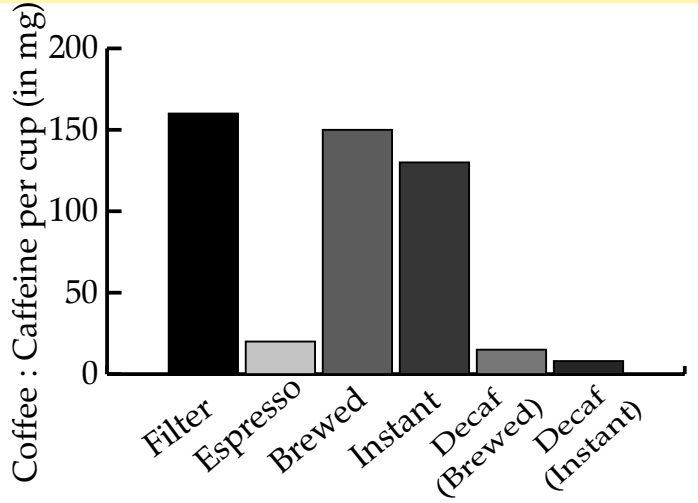Case Based Factual Passages (with Past Year Questions)

English Class 10

This question asked in [Delhi, 2016]

## The researchers, however, advise that one should consume coffee in moderation, because it can make you jittery  and cause stomach pains.#### (D) A Dietician

So, the correct answer is (D)

#### (D) coffee

So, the correct answer is (A)

#### (D) Hot chocolate

So, the correct answer is (C)

#### (D) Type 2

So, the correct answer is (D)

#### (D) similar

So, the correct answer is (B)

#### (D) no

So, the correct answer is (B)

#### (D) espresso

So, the correct answer is (C)

#### (D) 50 mg

So, the correct answer is (C)

#### (D) decaf instant

So, the correct answer is (D)

#### (D) none

So, the correct answer is (D)

#### (D) No effect

So, the correct answer is (C)

#### (D) quit the habit